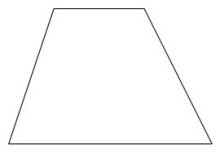# KLMN

In the trapezoid KLMN is given this informations:
1. segments KL and MN are parallel
2. segments KL and KM has same length
3. segments KN, NM and ML has same length.

Determine the size of the angle KMN.

Correct result:

α =  36 °

#### Solution:

|KL| = |KM| \ \\ |KN|=|NM|=|ML| \ \\ \alpha = &angle; KMN = &angle; NKM = &angle; MKL \ \\ \beta = &angle; KNM \ \\ \ \\ &angle; KML = \beta - \alpha \ \\ &angle; KLM = 180 ^\circ - \alpha - (\beta - \alpha) = 180 ^\circ - \beta \ \\ \ \\ \ \\ &Delta; KLM: \ \\ &angle; KML = &angle; KLM \ \\ \beta -\alpha = 180 ^\circ - \beta \ \\ \beta = 180 ^\circ + \alpha/2; \ \\ \ \\ &Delta; KMN: \ \\ 2\alpha + \beta = 180^\circ \ \\ 2\alpha + 90+ \alpha /2 = 180^\circ \ \\ 2.5 \alpha = 90^\circ \ \\ \ \\ \alpha = 36 ^\circWe would be pleased if you find an error in the word problem, spelling mistakes, or inaccuracies and send it to us. Thank you!Tips to related online calculators

#### You need to know the following knowledge to solve this word math problem:

We encourage you to watch this tutorial video on this math problem:

## Next similar math problems:

• Chocolate rollThe cube of 5 cm chocolate roll weighs 30 g. How many calories will contain the same chocolate roller of a prism shape with a length of 0.5 m whose cross section is an isosceles trapezoid with bases 25 and 13 cm and legs 10 cm. You know that 100 g of this
• Internal anglesThe ABCD is an isosceles trapezoid, which holds: |AB| = 2 |BC| = 2 |CD| = 2 |DA|: On its side BC is a K point such that |BK| = 2 |KC|, on its side CD is the point L such that |CL| = 2 |LD|, and on its side DA the point M is such that | DM | = 2 |MA|. DeteShow that the quadrilateral with vertices P1(0,1), P2(4,2) P3(3,6) P4(-5,4) has two right triangles.
• Children playgroundThe playground has the shape of a trapezoid, the parallel sides have a length of 36 m and 21 m, the remaining two sides are 14 m long and 16 m long. Determine the size of the inner trapezoid angles.
• MO - trianglesOn the AB and AC sides of the triangle ABC lies successive points E and F, on segment EF lie point D. The EF and BC lines are parallel and is true this ratio FD:DE = AE:EB = 2:1. The area of ABC triangle is 27 hectares and line segments EF, AD, and DB seg
• Cuboid face diagonalsThe lengths of the cuboid edges are in the ratio 1: 2: 3. Will the lengths of its diagonals be the same ratio? The cuboid has dimensions of 5 cm, 10 cm, and 15 cm. Calculate the size of the wall diagonals of this cuboid.
• Diagonals at right angleIn the trapezoid ABCD this is given: AB=12cm CD=4cm And diagonals crossed under a right angle. What is the area of this trapezoid ABCD?
• Rectangular trapezoidThe ABCD rectangular trapezoid with the AB and CD bases is divided by the diagonal AC into two equilateral rectangular triangles. The length of the diagonal AC is 62cm. Calculate trapezium area in cm square and calculate how many differs perimeters of the
• MO Z8–I–6 2018In the KLMN trapeze, KL has a 40 cm base and an MN of 16 cm. Point P lies on the KL line so that the NP segment divides the trapezoid into two parts with the same area. Find the length of the KP line.
• Trapezium diagonalsIt is given trapezium ABCD with bases | AB | = 12 cm, |CD| = 8 cm. Point S is the intersection of the diagonals for which |AS| is 6 cm long. Calculate the length of the full diagonal AC.
• Diagonal intersectisosceles trapezoid ABCD with length bases | AB | = 6 cm, CD | = 4 cm is divided into 4 triangles by the diagonals intersecting at point S. How much of the area of the trapezoid are ABS and CDS triangles?
• Trapezoid thirdsThe ABCD trapezoid with the parallel sides of the AB and the CD and the E point of the AB side if the segment DE divides the trapezoid into two parts with the same area. Find the length of the AE line segment.
• MastMast has 13 m long shadow on a slope rising from the mast foot in the direction of the shadow angle at angle 13.3°. Determine the height of the mast, if the sun above the horizon is at angle 45°12'.
• PrismRight angle prism, whose base is right triangle with leg a = 3 cm and hypotenuse c = 13 cm has same volume as a cube with an edge length of 3 dm. a) Determine the height of the prism b) Calculate the surface of the prism c) What percentage of the cube's s
• Diagonals in the diamondThe length of one diagonal in diamond is 24 cm greater than the length of the second diagonal and diamond area is 50 m2. Determine the sizes of the diagonals.
• Trapezium ABCDIn the figure, ABDC is a trapezium in which AB || CD. line segments RN and LM are drawn parallel to AB such that AJ=JK=KP. If AB=0.5m and AP=BQ=1.8m, find the lengths of AC, BD, RN and LM. angle D=angle C=60
• Isosceles trapezoidIn an isosceles trapezoid KLMN intersection of the diagonals is marked by the letter S. Calculate the area of trapezoid if /KS/: /SM/ = 2:1 and a triangle KSN is 14 cm2.